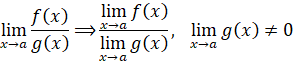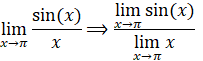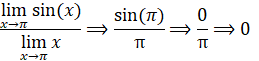It looks like you're using Internet Explorer 11 or older. This website works best with modern browsers such as the latest versions of Chrome, Firefox, Safari, and Edge. If you continue with this browser, you may see unexpected results.

# Math

Some of the content of this guide was modeled after a guide originally created by the Openstax and has been adapted for the GPRC Learning Commons in September 2021. The graphs are generated using Desmos. This work is licensed under a Creative Commons BY 4.0 International License.## Properties of Limits

Described in this section are the properties of limits, assuming that c is a constant and the following limits exist: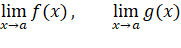Property Description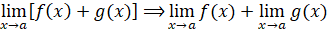The limit of a sum is equivalent to the sum of the limits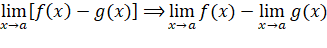The limit of a difference is equivalent to the difference of the limits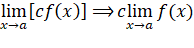The limit of a constant times a function is equivalent to the constant times the limit of the function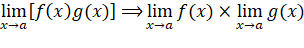The limit of a product is equivalent to the product of the limits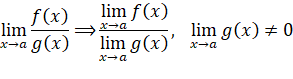The limit of a quotient is equivalent to the quotient of the limits

• Where the limit of the denominator is not zero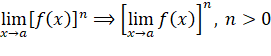The limit of a function to the power of n is equivalent to the finding the limit of the function and applying the power of n to the result

• Where n is greater than zero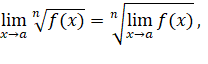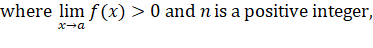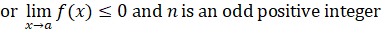The limit of a function to the root of n is equivalent to the finding the limit of the function and applying the root of n to the result

• Where the limit of the function is greater than zero and n is a positive integer

or

• Where the limit of the function is less than or equal to zero and n is an odd positive integer

Table 1: Properties of Limits

## Examples

Example 1: Limit of sums

The limit of sums is used when there are a combination of functions using addition.  The two separate parts of the function can be solved for the limit and those limits can be summed separately.For the function below, the two parts of the function can be separated and solved individually before recombining the results.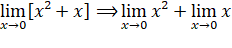Apply the basic limit laws and calculate the limit: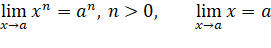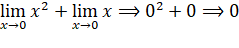Example 2: Limit of differences

The limit of differences is used when there are a combination of functions using subtraction.  The two separate parts of the function can be solved for the limit and those limits can be subtracted separately.For the below function, the two parts of the function can be separated and solved individually before subtracting the results.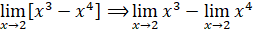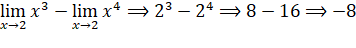Example 3: Limit of constant times a function

As described, if a function consists of a constant as a multiplier, the constant can be removed and the limit of the function can be solved with the multiplier applied afterwards.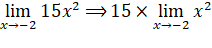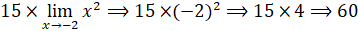Example 4: Limit of products

The limit of products is used when there are a combination of functions using multiplication.  The two separate parts of the function can be solved for the limit and those limits can be multiplied separately.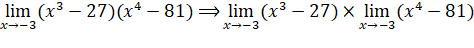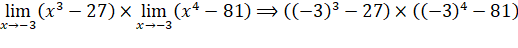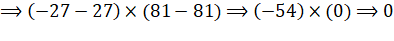Example 5: Limit of quotients

The limit of quotients is used when there are a combination of functions using division.  The two separate parts of the function can be solved for the limit and those limits can be divided separately, if the limit of the denominator does not equal zero.